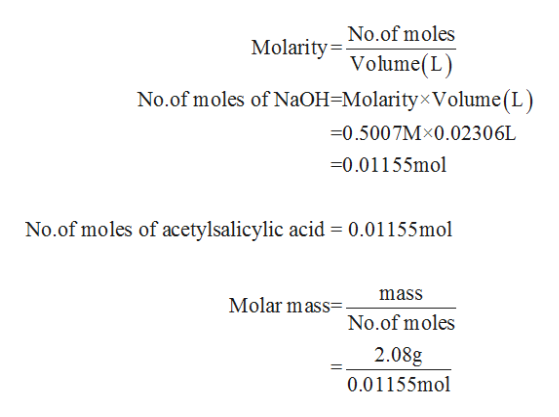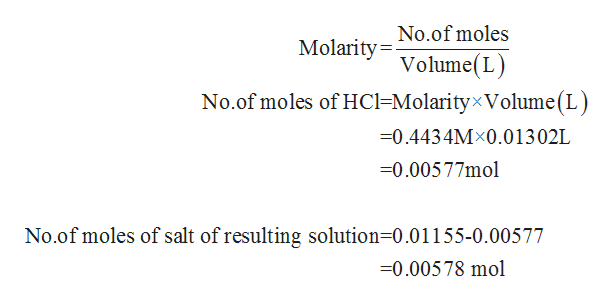# The active ingredient in aspirin is acetylsalicylic acid. A 2.08 g sample of acetylsalicylic acid required 23.06 mL of 0.5007 M NaOH for complete reaction. Addition of 13.02 mL of 0.4434 M HCl to the flask containing the aspirin and the sodium hydroxide produced a mixture with pH = 3.48. Find the molar mass (in g/mol) of acetylsalicylic acid and its Ka value. Acetylsalicylic acid is a monoprotic acid.

Question
471 views

The active ingredient in aspirin is acetylsalicylic acid. A 2.08 g sample of acetylsalicylic acid required 23.06 mL of 0.5007 M NaOH for complete reaction. Addition of 13.02 mL of 0.4434 M HCl to the flask containing the aspirin and the sodium hydroxide produced a mixture with pH = 3.48. Find the molar mass (in g/mol) of acetylsalicylic acid and its Ka value. Acetylsalicylic acid is a monoprotic acid.

check_circle

Step 1

Number of moles of acetylsalicylic acid and NaOH will be equal since one carboxylic acid group is present in acetylsalicylic acid.help_outlineImage TranscriptioncloseNo.of moles Molarity= Volume(L) No.of moles of NaOH=Molarity×Volume(L) =0.5007M×0.02306L =0.01155mol No.of moles of acetylsalicylic acid = 0.01155mol mass Molar mass=. No.of moles 2.08g 0.01155mol fullscreen
Step 2

Number of moles of HCl can be determin...help_outlineImage TranscriptioncloseNo.of moles Molarity=- Volume(L) No.of moles of HC1=Molarity×Volume(L) =0.4434M×0.01302L =0.00577mol No.of moles of salt of resulting solution=0.01155-0.00577 =0.00578 mol fullscreen

### Want to see the full answer?

See Solution

#### Want to see this answer and more?

Solutions are written by subject experts who are available 24/7. Questions are typically answered within 1 hour.*

See Solution
*Response times may vary by subject and question.
Tagged in

### General Chemistry